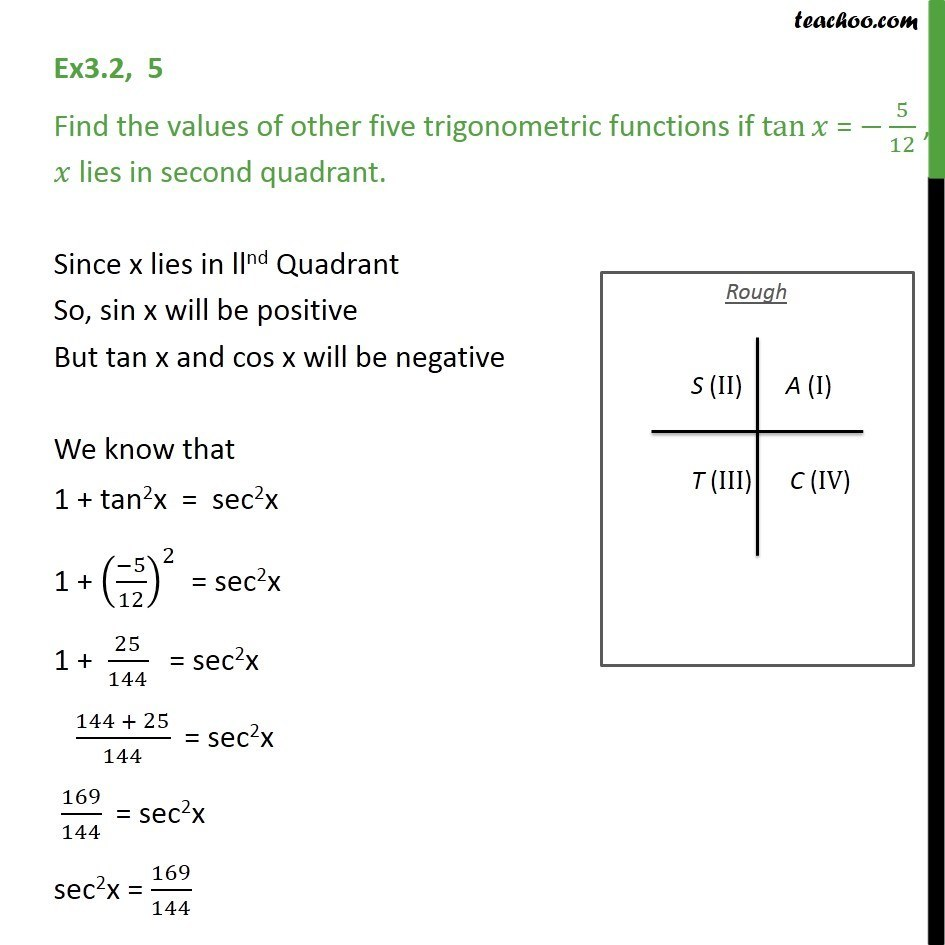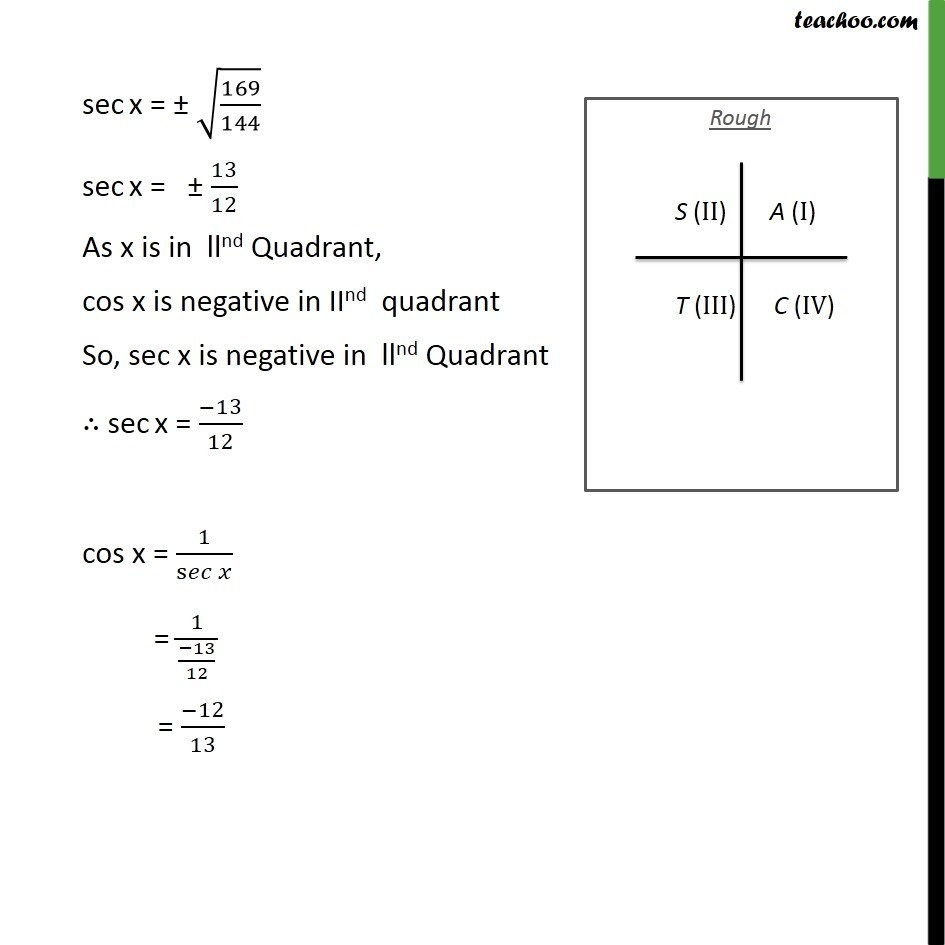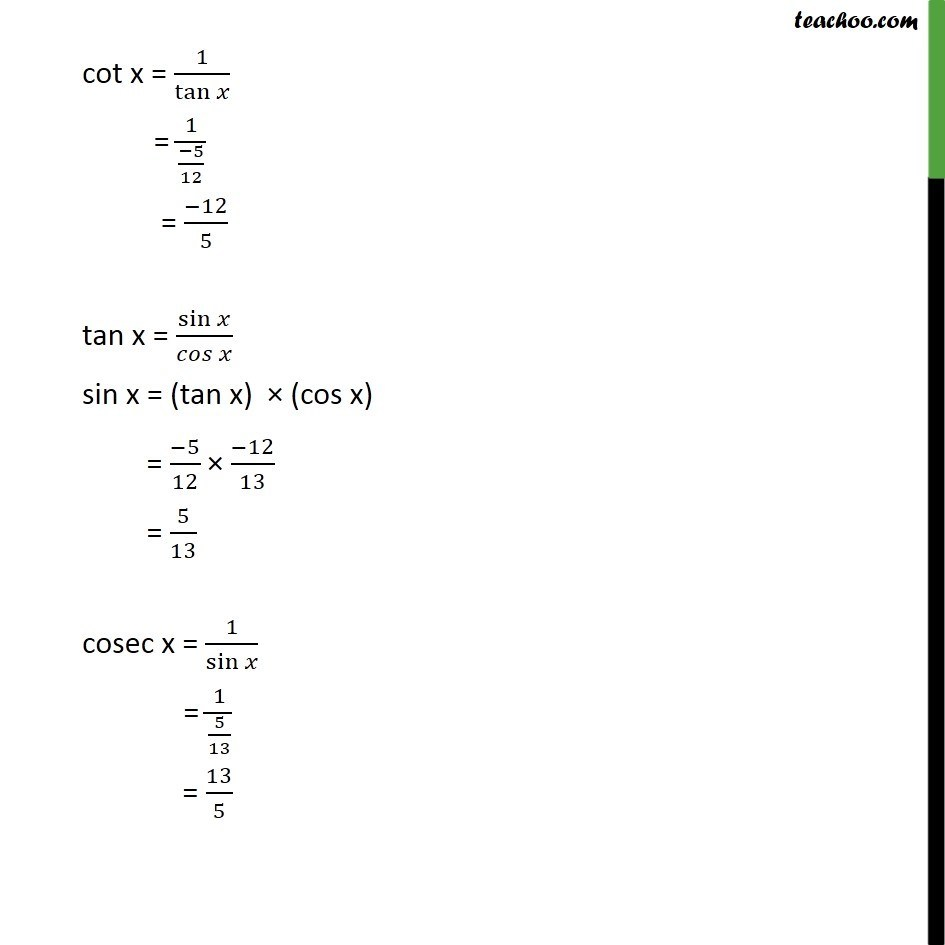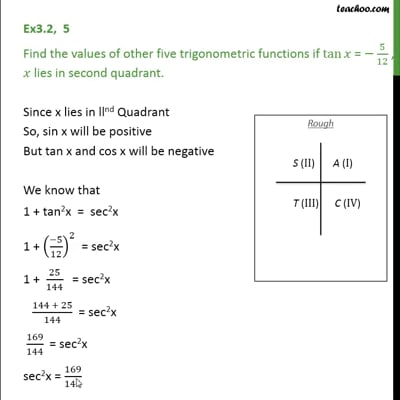Ex 3.2

Chapter 3 Class 11 Trigonometric Functions (Term 2)
Serial order wiseThis video is only available for Teachoo black users

### Transcript

Ex3.2, 5 Find the values of other five trigonometric functions if tan = 5/12 , lies in second quadrant. Since x lies in llnd Quadrant So, sin x will be positive But tan x and cos x will be negative We know that 1 + tan2x = sec2x 1 + (( 5)/12)^2 = sec2x 1 + 25/144 = sec2x (144 + 25)/144 = sec2x 169/144 = sec2x sec2x = 169/144 sec x = (169/144) sec x = 13/12 As x is in llnd Quadrant, cos x is negative in IInd quadrant So, sec x is negative in llnd Quadrant sec x = ( 13)/12 cos x = 1/s = 1/(( 13)/12) = ( 12)/13 cot x = 1/tan = 1/(( 5)/12) = ( 12)/5 tan x = sin /( ) sin x = (tan x) (cos x) = ( 5)/12 ( 12)/13 = 5/13 cosec x = 1/sin = 1/(5/13) = 13/5